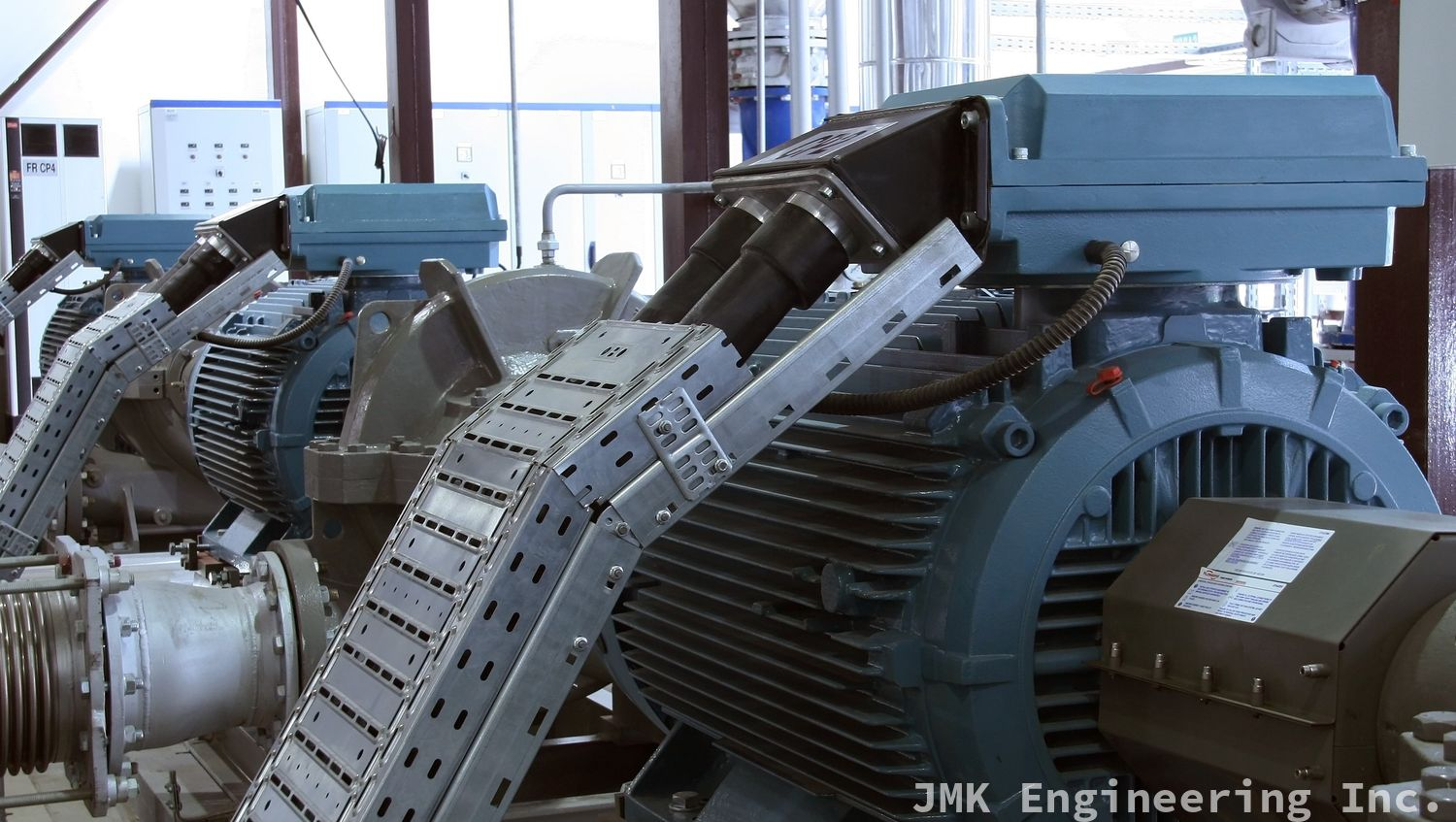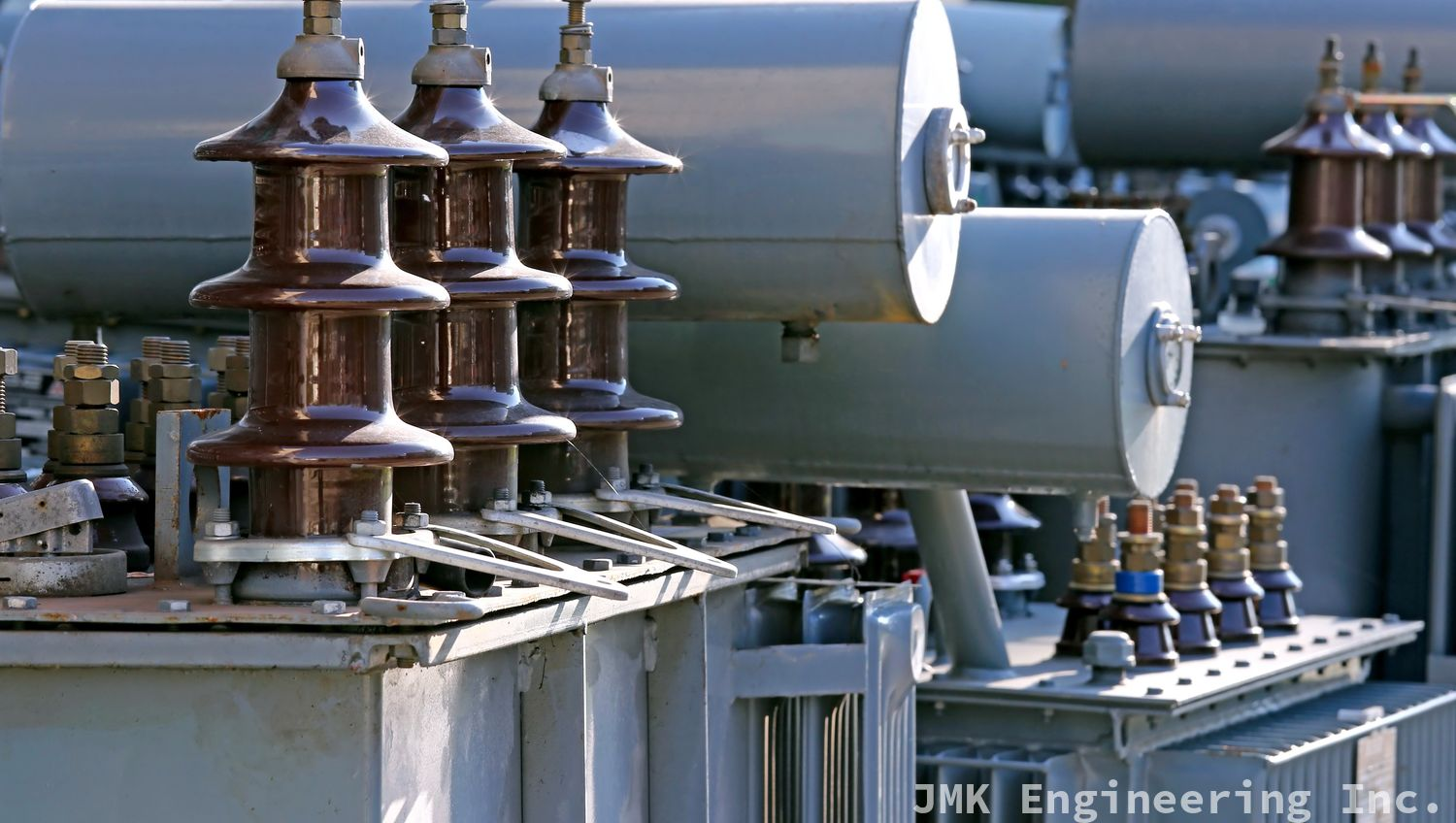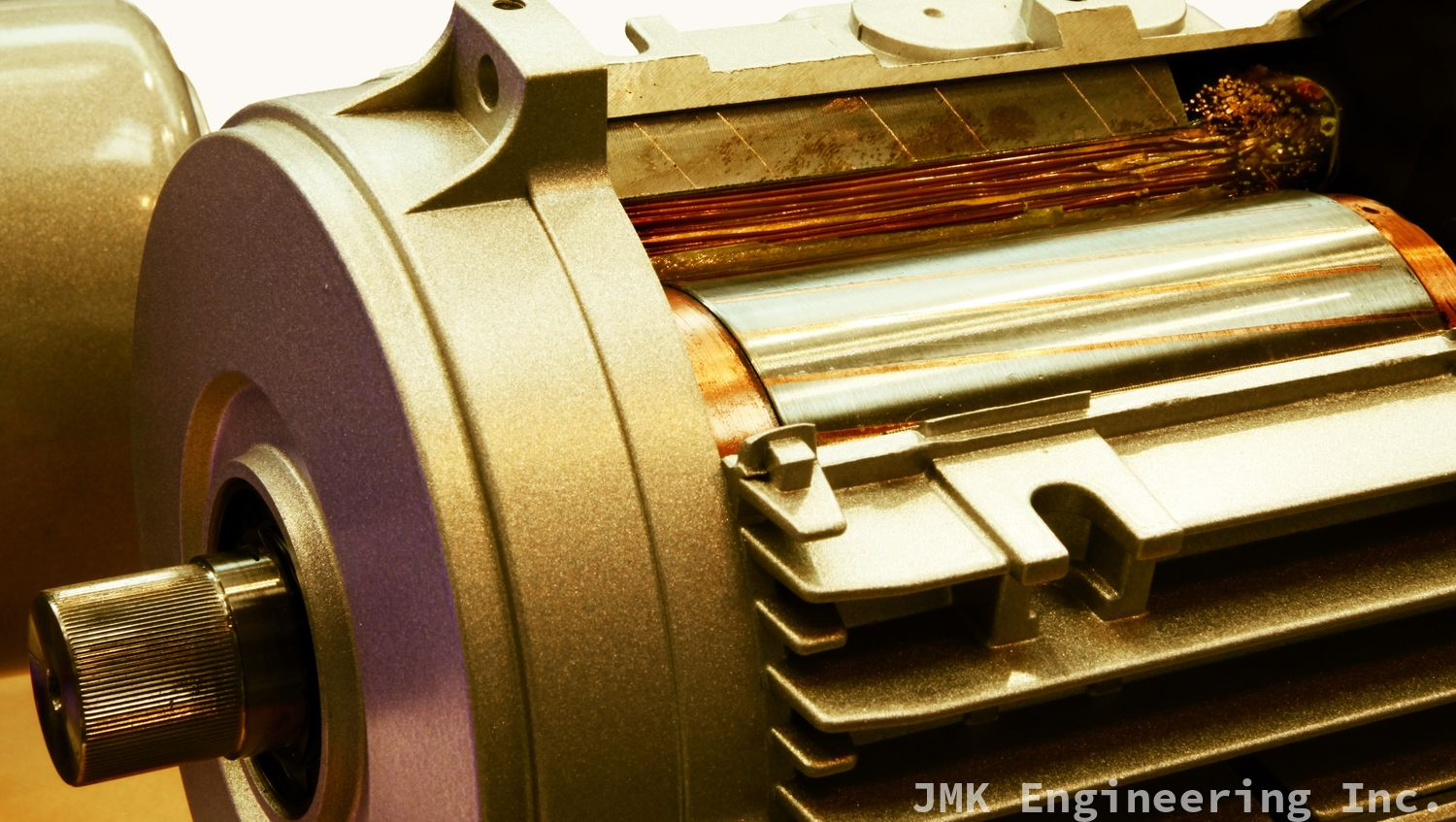# How Induction Machines Work

Published:There are two main types of induction machines used to transmit electrical energy and perform work: transformers, and AC induction motors. In order to understand how they work, first you need to understand the principle of induction.

## Induction

Induction is the process by which a magnetic field can induce current in a wire. The easiest way to test this is to slide a bar magnet through a coil of copper wire and measure the current through the wire. As the magnet passes through the wire, an electric current is generated.

## Induction Machines: TransformersTransformers use the property of induction to change voltage and current values in an electric circuit without altering the source of the electricity. At the lowest level, a transformer is made of a metal core (usually iron) with two copper windings (wire tightly wrapped around the transformer core). These windings are referred to as primary windings (where the current enters the transformer from the source) and the secondary windings (where the current leaves the transformer to go farther down the power line). As current travels through the wires of the primary winding, it generates a magnetic field that moves through the transformer core. The core is shaped like a hoop: when the magnetic field reaches the part of the core that the secondary winding is wrapped around, the magnetic field induces an electric current in the secondary winding. The magnitude of this current is proportional to the number of turns of wiring in the winding.

## Induction Machines: Motors

The most common type of induction machine is a three-phase, squirrel cage induction motor. An induction motor consists of two parts: a stator and a rotor.### Stator

The stator of an induction motor is the non-moving part of the motor. It consists of three pairs of "poles" for the three phases of AC current. The poles are arranged so that when the phases of the oscillating current flow through them, they generate a rotating magnetic field.

### Rotor

The rotor of the induction motor is shaped like a squirrel cage, with a number of metal bars joined electrically at either end by two metal rings (which short circuits the rotor).

## How It Works

The rotating magnetic field generated by the stator is moving relative to the rotor. The rotor wants to have the same rotating magnetic field as the stator, so a current is induced in the rotor and it is forced to move to try and line up the magnetic field of the rotor with the magnetic field of the stator. If the rotor's field lined up it would reach "synchronous speed" and stop moving (because the two fields would be in equilibrium). For this reason, the rotor will always move slower than the synchronous speed. The difference between the actual speed of a motor and the synchronous speed of the AC current is called slip.

## Torque and SpeedIn order for an induction motor to be useful, it has to drive a load at a certain speed. In order to move the load, the motor must generate a certain amount of torque. The torque generated by the motor is proportional to the amount of current that the motor is drawing from its source: more current means more torque. However, the speed of the motor is proportional to the voltage, and voltages at the source are normally fixed. What this means is that if your motor load requires high torque, the high current draw will force the voltage down and this will result in a slow motor.

This is how motor starting works, and most motors are designed to draw a lot of current when they start, in order to generate the torque necessary to move what is initially a static load. As the load starts moving, the torque required to move it is reduced and the motor can speed up. It continues on like this until the motor reaches its operational speed. In the case where for some reason voltage drops, the strain on the motor (from slower speed, higher torque, and higher current) can cause the motor to burn out. You can find out more about how voltage drop affects induction machines here.

When you break them down, induction machines aren't nearly as complicated as they seem! As always, thanks for reading!